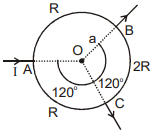Select Chapter Topics:Which one of the following gives the value of the magnetic field according to Biot-Savart’s law?

1. $\frac{\mathrm{i}∆\mathrm{lsin}\left(\mathrm{\theta }\right)}{{\mathrm{r}}^{2}}$

2. $\frac{{\mathrm{\mu }}_{0}}{4\mathrm{\pi }}\frac{\mathrm{i}∆\mathrm{lsin}\left(\mathrm{\theta }\right)}{\mathrm{r}}$

3. $\frac{{\mathrm{\mu }}_{0}}{4\mathrm{\pi }}\frac{\mathrm{i}∆\mathrm{lsin}\left(\mathrm{\theta }\right)}{{\mathrm{r}}^{2}}$

4. $$\frac{\mu_0}{4 \pi} \mathrm{i} \Delta \mathrm{l} \sin (\theta)$$Subtopic:  Biot-Savart Law |
89%
From NCERT
To view explanation, please take trial in the course.
NEET 2023 - Target Batch - Aryan Raj Singh
Hints
To view explanation, please take trial in the course.
NEET 2023 - Target Batch - Aryan Raj Singh

To maximise the magnetic field caused by a small element of a current-carrying conductor at a point, the angle between the element and the line connecting the element to the point P must be:

1. 0º

2. 90º

3. 180º

4. 45ºSubtopic:  Biot-Savart Law |
87%
From NCERT
To view explanation, please take trial in the course.
NEET 2023 - Target Batch - Aryan Raj Singh
Hints
To view explanation, please take trial in the course.
NEET 2023 - Target Batch - Aryan Raj Singh

An element $$\Delta l=\Delta x \hat{i}$$ is placed at the origin and carries a large current of $$I=10$$ A (as shown in the figure). What is the magnetic field on the y-axis at a distance of $$0.5$$ m? ($$\Delta x=1~\mathrm{cm}$$)1. $$6\times 10^{-8}~\mathrm{T}$$
2. $$4\times 10^{-8}~\mathrm{T}$$
3. $$5\times 10^{-8}~\mathrm{T}$$
4. $$5.4\times 10^{-8}~\mathrm{T}$$Subtopic:  Biot-Savart Law |
79%
To view explanation, please take trial in the course.
NEET 2023 - Target Batch - Aryan Raj Singh
Hints
To view explanation, please take trial in the course.
NEET 2023 - Target Batch - Aryan Raj Singh

A straight wire carrying a current of 12 A is bent into a semi-circular arc of radius 2.0 cm as shown in the figure. Considering the magnetic field B at the centre of the arc, what will be the magnetic field due to the straight segments?Subtopic:  Biot-Savart Law |
76%
To view explanation, please take trial in the course.
NEET 2023 - Target Batch - Aryan Raj Singh
Hints

Which one of the following expressions represents Biot-Savart's law? Symbols have their usual meanings.

1. $\stackrel{}{\stackrel{\to }{dB}}=\frac{{\mu }_{0}\mathrm{I}\left(\stackrel{}{\stackrel{\to }{dl}}×\stackrel{^}{r}\right)}{4\mathrm{\pi }{\left|\stackrel{\to }{\mathrm{r}}\right|}^{3}}$

2. $\stackrel{}{\stackrel{\to }{dB}}=\frac{{\mu }_{0}\mathrm{I}\left(\stackrel{}{\stackrel{\to }{dl}}×\stackrel{^}{r}\right)}{4\mathrm{\pi }{\left|\stackrel{\to }{\mathrm{r}}\right|}^{2}}$

3. $\stackrel{}{\stackrel{\to }{dB}}=\frac{{\mu }_{0}\mathrm{I}\left(\stackrel{}{\stackrel{\to }{dl}}×\stackrel{}{\stackrel{\to }{r}}\right)}{4\mathrm{\pi }{\left|\stackrel{\to }{\mathrm{r}}\right|}^{3}}$

4. $\stackrel{}{\stackrel{\to }{dB}}=\frac{{\mu }_{0}\mathrm{I}\left(\stackrel{}{\stackrel{\to }{dl}}.\stackrel{}{\stackrel{\to }{r}}\right)}{4\mathrm{\pi }{\left|\stackrel{\to }{\mathrm{r}}\right|}^{3}}$Subtopic:  Biot-Savart Law |
67%
Hints

A long wire carrying a steady current is bent into a circular loop of one turn. The magnetic field at the centre of the loop is B. It is then bent into a circular coil of n turns. What will the magnetic field be at the centre of this n-turn coil?

1.  nB

2.  n2B

3.  2nB

4.  2n2BSubtopic:  Magnetic Field due to various cases |
83%
From NCERT
NEET - 2016
To view explanation, please take trial in the course.
NEET 2023 - Target Batch - Aryan Raj Singh
Hints
To view explanation, please take trial in the course.
NEET 2023 - Target Batch - Aryan Raj Singh

The magnetic induction at point P, which is 4 cm from a long current-carrying wire is 10-8 Tesla. What would be the field of induction at a distance of 12 cm from the same current?

 1 3.33 x 10-9 Tesla 2 1.11 x 10-4 Tesla 3 3 x 10-3 Tesla 4 9 x 10-2 TeslaSubtopic:  Magnetic Field due to various cases |
80%
From NCERT
To view explanation, please take trial in the course.
NEET 2023 - Target Batch - Aryan Raj Singh
Hints
To view explanation, please take trial in the course.
NEET 2023 - Target Batch - Aryan Raj Singh

Two similar coils of radius R are lying concentrically with their planes at right angles to each other. The currents flowing in them are I and 2I, respectively. What will be the resultant magnetic field induction at the centre?

1. $\frac{\sqrt{5}{\mu }_{0}I}{2R}$

2. $\frac{3{\mu }_{0}I}{2R}$

3. $\frac{{\mu }_{0}I}{2R}$

4. $\frac{{\mu }_{0}I}{R}$Subtopic:  Magnetic Field due to various cases |
79%
From NCERT
AIPMT - 2012
To view explanation, please take trial in the course.
NEET 2023 - Target Batch - Aryan Raj Singh
Hints
To view explanation, please take trial in the course.
NEET 2023 - Target Batch - Aryan Raj Singh

The resistances of three parts of a circular loop are as shown in the figure. What will be the magnetic field at the centre of O
(current enters at A and leaves at B and C as shown)?1.  $\frac{{\mathrm{\mu }}_{0}\mathrm{I}}{6\mathrm{a}}$

2.  $\frac{{\mathrm{\mu }}_{0}\mathrm{I}}{3\mathrm{a}}$

3.  $\frac{2{\mathrm{\mu }}_{0}\mathrm{I}}{3\mathrm{a}}$

4.  0Subtopic:  Magnetic Field due to various cases |
81%
From NCERT
To view explanation, please take trial in the course.
NEET 2023 - Target Batch - Aryan Raj Singh
Hints
To view explanation, please take trial in the course.
NEET 2023 - Target Batch - Aryan Raj Singh

Which of the following graphs correctly represents the variation of magnetic field induction with distance due to a thin wire carrying current?

 1234Subtopic:  Magnetic Field due to various cases |
79%
From NCERT
To view explanation, please take trial in the course.
NEET 2023 - Target Batch - Aryan Raj Singh
Hints